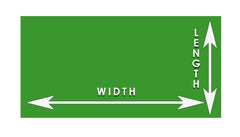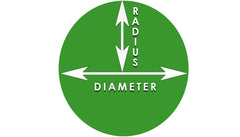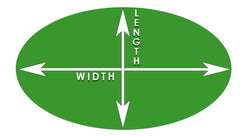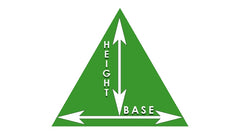# How to Measure your Turf area (meters squared)

Measure the proposed spray area in square metres before starting your spray treatment process.
If you have an irregular shaped turf area, draw it up as accurately as you can and then divide it into any combination of the shapes below.
Calculate the area of each shape and then add these amounts together to determine the final area.
When the final area is determined you can then calculate your rate of product to be sprayed per 100m2.

 RECTANGLE OR SQUARE AREASIf you have a square of rectangle lawn area you are very fortunate because it won’t get any easier than this. Simply multiple the length by the width to reach your total.For example:Length = 7mWidth = 5m7m x 5m = 35m2 CIRCLE AREASMeasure the radius, then multiply the radius by itself and then by 3.14 (π).For example:Radius = 55m x 5m x 3.14 = 78.5m2 OVAL AREASMeasure the length and width in meters, then multiply the length by 0.80 before multiplying the result by width.For example:Length = 18mWidth = 10m18m x 0.80 = 14.4m14.4m x 10m = 144m2 TRIANGLE AREASMeasure the base and height in meters, then multiply the base by 0.5 before multiplying that by the height. For example: Base = 12m Height = 14m 12 x 0.5 x 14 = 84m2

Draw a diagram and block areas off and measure, then add your measure areas in square meters (m2) up together to get your total area.

For example;